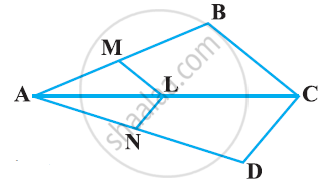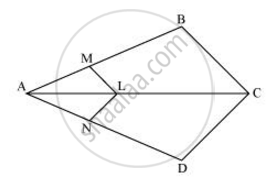# In the following figure, if LM || CB and LN || CD, prove that (AM)/(AB)=(AN)/(AD) - Mathematics

In the following figure, if LM || CB and LN || CD, prove that (AM)/(AB)=(AN)/(AD)#### SolutionIn the given figure, LM || CB

By using basic proportionality theorem, we obtain

(AM)/(AB) = (AL)/(AC)  ........(i)

Similary, LN || CD

:.(AN)/(AD)=(AL)/(AC).........(ii)

From (i) and (ii), we obtain

(AM)/(AD) = (AN)/(AD)

Concept: Similarity of Triangles
Is there an error in this question or solution?

#### APPEARS IN

NCERT Class 10 Maths
Chapter 6 Triangles
Exercise 6.2 | Q 3 | Page 128